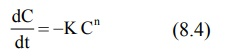# Rate, Rate Constants and Orders of Reactions

| Home | | Biopharmaceutics and Pharmacokinetics |

## Chapter: Biopharmaceutics and Pharmacokinetics : Pharmacokinetics Basic Considerations

Pharmacokinetics is the mathematical analysis of processes of ADME.

Rate, Rate Constants and Orders of Reactions

Pharmacokinetics is the mathematical analysis of processes of ADME. The movement of drug molecules from the site of application to the systemic circulation, through various barriers, their conversion into another chemical form and finally their exit out of the body can be expressed mathematically by the rate at which they proceed, the order of such processes and the rate constants.

The velocity with which a reaction or a process occurs is called as its rate. The manner in which the concentration of drug (or reactants) influences the rate of reaction or process is called as the order of reaction or order of process. Consider the following chemical reaction:

Drug A Drug B               (8.1)

The rate of forward reaction is expressed as –

-dA/dt                  (8.2)

Negative sign indicates that the concentration of drug A decreases with time t. As the reaction proceeds, the concentration of drug B increases and the rate of reaction can also be expressed as:

dB/dt          (8.3)

Experimentally, the rate of reaction is determined by measuring the decrease in concentration of drug A with time t.

If C is the concentration of drug A, the rate of decrease in C of drug A as it is changed to B can be described by a general expression as a function of time t.where, K = rate constant

n = order of reaction

If n = 0, its a zero-order process, if n = 1, it is a first-order process and so on. The three commonly encountered rate processes in a physiological system are —

·            Zero-order process

·            First-order process

·            Mixed-order process.

The pharmacokinetics of most drugs can be adequately described by zero- and first-order processes of which the latter are more important.